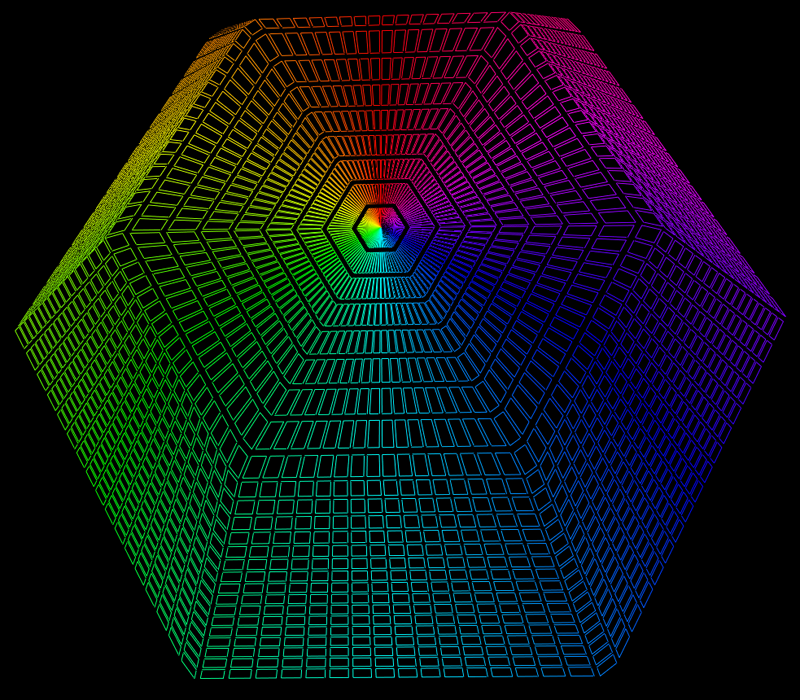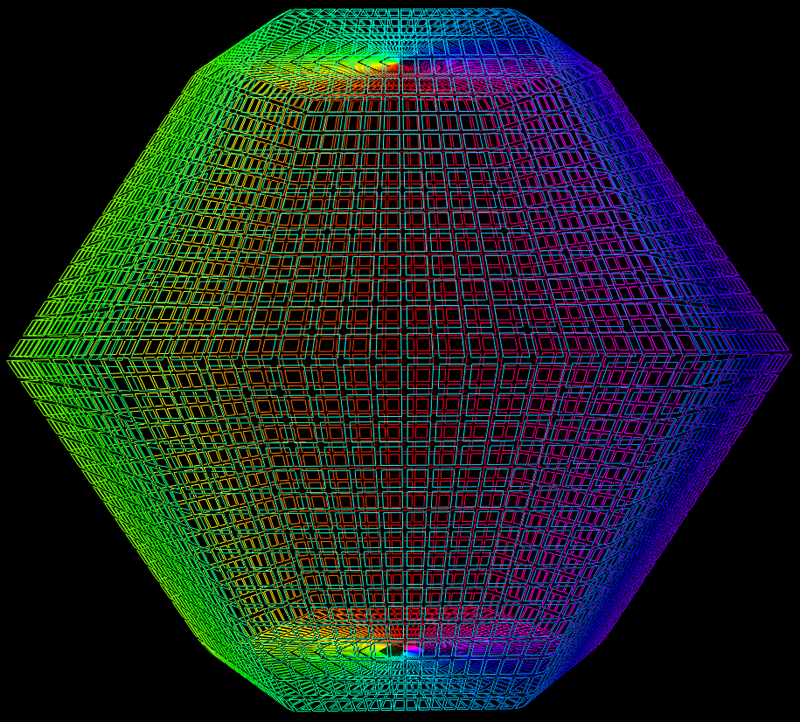# Hexagonal Drum

Graphics by Paul Bourke
July 2012

Equations by Roger Bagula

H(t) = | cos(t) | + | cos(t+2pi/3) |
I(t) = | cos(t+2pi/3) | + | cos(t-2pi/3) |
J(t) = | cos(t-2pi/3) | + | cos(t) |
x = 16 * cos(u) sin(v) / [(H(u) + I(u) + J(u))(H(v) + I(v) + J(v))]
y = 16 * sin(u) sin(v) / [(H(u) + I(u) + J(u))(H(v) + I(v) + J(v))]
z = 4 * cos(u) / [H(u) + I(u) + J(u)]
0 <= u <= 2 pi, 0 < v < pi
Scaling above gives a unit height drum.